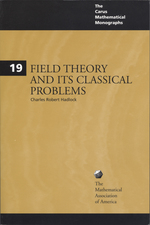Have library access? Log in through your library# Field Theory and its Classical Problems

CHARLES ROBERT HADLOCK
Volume: 19
Copyright Date: 1975
Edition: 1
Pages: 340
https://www.jstor.org/stable/10.4169/j.ctt5hh8wp

## Table of Contents

1. Front Matter
(pp. i-viii)
2. PREFACE
(pp. ix-xiv)
Charles R. Hadlock
3. Table of Contents
(pp. xv-xvi)
4. INTRODUCTION
(pp. 1-8)

Mathematical knowledge has undergone explosive growth in the past few hundred years. No doubt, a significant portion of this growth is due to the usefulness of mathematics as a tool in many fields. However, probably the greatest single stimulus to the development of this subject is its natural intellectual appeal—the challenge of a hard problem and the satisfaction of resolving it. For centuries these factors have motivated the efforts of mathematicians.

Problems which are simple to state and understand are quite reasonably often the most attractive. It is not uncommon for such problems to stimulate extensive research by many...

5. CHAPTER 1 THE THREE GREEK PROBLEMS
(pp. 9-58)

An important step in the solution of the classical Greek construction problems will be taken in this section, where we shall answer the question: Given a line segment of length 1 in the plane, for what values ofacan we construct a segment of lengtha?As usual in geometry, we shall always consider lengths to be non-negative. To begin, naturally, we need to take a careful look at the rules which govern the construction, where by “construction” we refer to a finite sequence of operations with a compass and an unmarked straightedge. The individual operations which may be...

6. CHAPTER 2 FIELD EXTENSIONS
(pp. 59-122)

In the last chapter, we saw how certain classical geometric construction problems depended for their solution on the nature of the roots of certain polynomials. We shall take another look at these problems later in this chapter. In the next chapter, we shall look at another famous problem, namely, the problem of trying to find analogues of the quadratic formula for the roots of polynomials of degree greater than 2. Since all these investigations deal with polynomials, we need first to learn more about the ‘arithmetic’ of polynomials.

Given a field F, we denote by F[x] the set of all...

7. CHAPTER 3 SOLUTION BY RADICALS
(pp. 123-180)

As every student of mathematics knows, the solutions to the quadratic equation$a{x^2} + bx + c = 0$are given by the formula$x = ( - b \pm \sqrt {{b^2} - 4ac} )/2a$. In this chapter we essentially ask: To what extent can similar formulas be found for polynomials of higher degree?

To begin, the term “similar formula” needs to be given precise meaning. For our purposes, we shall consider a formula to be similar if it involves only a sequence of rational operations and the extraction of roots (of any integral order), beginning with the coefficients. Since, beginning with any nonzero coefficient, any rational number may be computed by a sequence of rational...

8. CHAPTER 4 POLYNOMIALS WITH SYMMETRIC GROUPS
(pp. 181-220)

The proof of Abel’s Theorem showed that for every n ≥ 5 there exists a polynomialfof degreenover Q such thatat least oneroot offcannot be expressed in radicals. A natural question is whether a stronger result may actually be true, namely: For each value of n ≥ 5, does there exist a polynomialfof degreenover Q such that none of the roots offcan be expressed in radicals? As was pointed out just after the proof of Abel’s Theorem, the quintic polynomial used there actually has this stronger property....

9. SOLUTIONS TO THE PROBLEMS
(pp. 221-318)
10. INDEX
(pp. 319-323)